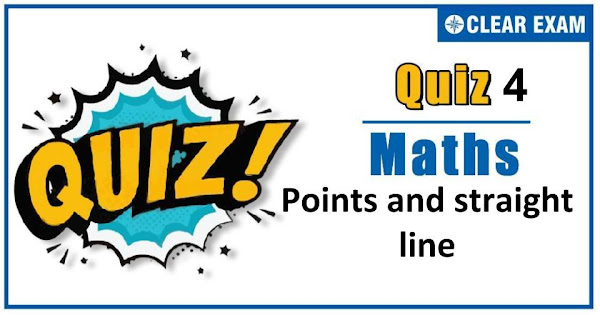## [LATEST]\$type=sticky\$show=home\$rm=0\$va=0\$count=4\$va=0

Dear Readers, As per analysis for previous years, it has been observed that students preparing for JEE MAINS find Mathematics out of all the sections to be complex to handle and the majority of them are not able to comprehend the reason behind it. This problem arises especially because these aspirants appearing for the examination are more inclined to have a keen interest in Mathematics due to their ENGINEERING background.
Furthermore, sections such as Mathematics are dominantly based on theories, laws, numerical in comparison to a section of Engineering which is more of fact-based, Physics, and includes substantial explanations. By using the table given below, you easily and directly access to the topics and respective links of MCQs. Moreover, to make learning smooth and efficient, all the questions come with their supportive solutions to make utilization of time even more productive. Students will be covered for all their studies as the topics are available from basics to even the most advanced.
.

Q1.  The lines p(p2+1)x-y+q=0 and (p2+1)2 x+(p2+1)y+2q=0 are perpendicular to a common line for
•  Exactly one value of p
•  Exactly two values of p
•  More than two values of p
•  No value of p
Solution
(a) Line perpendicular to same line are parallel to each other. ∴ -p(p2+1)=p2+1 ⇒ p=-1 ∴ There is exactly one value of p.
Q2.The orthocentre of the triangle formed by (0,0),(8,0),(4,6) is
•  (4,8/3)
•  (3,4)
•   (4,3)
•  (-3,4)
Solution
(a) Let the vertices of the triangle be O(0,0),A(8,0) and B(4,6). The equation of an altitude through O and perpendicular to AB is y=2/3 x and the equation of an altitude through A(8,0) and perpendicular to OB is 3 y=-2 x+16. These altitudes intersect at (4,8/3)
Q3.  The equation 8x2+8xy+2y2+26x+13y+15=0 represents a pair of straight lines. The distance between then is
•  7/√5
•  7/(2√5)
•  √7/5
•  None of these
Solution
(b) Now, h2-ab=42-8(2)=16-16=0 The required distance between the parallel straight lines =2√((169-120)/80)=(2×7)/(4√5)=7/(2√5)

Q4. The angle between the lines in x2-xy-6y2-7x+31y-18=0 is
•  60°
•  45°
•  30°
•  90°
Solution
b) Here,h=-1/2,a=1,b=-6 ∴tan⁡= |2 √(1/4+6)/(1-6)|=(2√(25/4))/(-5)=|-1| ∴ θ=tan-1 (1)=45°
Q5.The ends of the base of an isosceles triangle are at (2a,0) and (0,a). The equation of one side is x=2a. The equation of the
other side is
•  x+2y-a=0
•  x+2y = 2a
•  3x + 4y - 4a=0
•  3x - 4y+ 4a=0
Solution
d) Let C(2a,λ) be the third vertex, Clearly, AC=λ ∴BC=AC⇒√(4a2+(λ-a)2 )=λ⇒λ=5a/2 Thus, the coordinates of C are (2a,5a/2) Hence, the equation of BC is y-a=(5a/2-a)/(2a-0)(x-0) ⇒y-a=3/4 x ⇒3x-4y+4a=0
Q6. The diagonals of the parallelogram whose sides are lx+my+n=0,lx+my+n'=0,mx+ly+n=0,mx+ly+n'=0 include an angle
•  π/3
•  π/2
•  tan-1⁡((l2-m2)/(l2+m2))
•  tan-1⁡((2 lm)/(l2+m2))
Solution
b) Since the distance between the parallel lines lx+my+n=0 and lx+my+n^'=0 is same as the distance between parallel lines mx+ly+n=0 and mx+ly+n'=0. Therefore, the parallelogram is a rhombus. Also, the diagonals of a rhombus are at right angles. Therefore, the required angle is a right angle.
Q7.The inclination of the straight line passing through the point (-3,6) and the mid point of the line joining the points
(4,-5) and (-2,9) is
•   π/4
•  π/6
•  π/3
•  3π/4
Solution
d) Mid point of the line joining the points (4,-5) and (-2,9) is ((4-2)/2,(-5+9)/2) ie,(1,2) ∴ Inclination of straight line passing through point (-3,6) and mid point (1, 2) is m=(2-6)/(1+3)=(-4)/4=-1 ∴tan⁡θ=-1 ⇒ θ=3π/4
Q8.The distance of the line 2x-3y=4 from the point (1, 1) measured parallel to the line x+y=1, is
•  √2
•  5/√2
•  1/√2
•  6
Solution
(a) ∵ The slope of line x+y=1 is -1 ∴ It makes an angle of 135° with x-axis The equation of line passing through (1, 1) and making an angle of 135° is (x-1)/cos⁡135° =(y-1)/sin⁡135° =r ⇒(x-1)/(-1/√2)=(y-1)/(1/√2)=r Coordinates of any point on this line are (1-r/√2,1+r/√2) If this point lies on 2x-3y=4, then 2(1-r/√2)-3(1+r/√2)=4 ⇒2-2r/√2-3-3r/√2=4 ⇒5r/√2=-5 ⇒r=√2 (neglect negative sign)
Q9. The distance between the pair of lines given by x2+y2+2xy-8ax-8ay-9a2=0 is
•  2√5 a
•  10√a
•  10a
•  5√2 a
Solution
(d) We have, x2+y2+2xy-8ax-8ay-9a2=0 ⇒(x+y)2-8a(x+y)-9a2=0 ⇒(x+y-9a)(x+y+a)=0 ⇒x+y-9a=0,x+y+a=0 Clearly, these lines are parallel. The distance d between these lines is d=(|a-(-9a)|)/√(12+12 )=5√(2 ) a
Q10. Consider the fourteen lines in the plane given by y=x+r,y=-x+r, where r∈{0,1,2,3,4,5,6}. The number of squares formed by these lines, whose sides are of length √2, is
•   9
•  16
•  25
•  36
Solution
c) The given lines are perpendicular to each other. ∴Perpendicular distance=|r1-r2 |/√2=√2 ⇒ r1-r2=2 The difference between the y-intercepts =2 This can happen for five combinations {(0, 2), (1, 3), (2, 4), (3, 5), (4, 6)}. The difference between the x-intercepts =2 This can happen for five combinations. Hence, total number of squares =5×5=25#### Written by: AUTHORNAME

AUTHORDESCRIPTION## Want to know more

Please fill in the details below:

## Latest NEET Articles\$type=three\$c=3\$author=hide\$comment=hide\$rm=hide\$date=hide\$snippet=hide

Name

ltr
item
BEST NEET COACHING CENTER | BEST IIT JEE COACHING INSTITUTE | BEST NEET, IIT JEE COACHING INSTITUTE: Point and Straight Line Quiz-4
Point and Straight Line Quiz-4
https://1.bp.blogspot.com/-ANFbF28WQEE/X6QiMDGEf6I/AAAAAAAAKQM/LyZ3jyq5Qw8UNaS4qll1Rr_V6RMTV5HAACLcBGAsYHQ/s600/Copy%2Bof%2BQuiz%2BImage%2BTemplate%2B%25281%2529%2B%25283%2529.jpg
https://1.bp.blogspot.com/-ANFbF28WQEE/X6QiMDGEf6I/AAAAAAAAKQM/LyZ3jyq5Qw8UNaS4qll1Rr_V6RMTV5HAACLcBGAsYHQ/s72-c/Copy%2Bof%2BQuiz%2BImage%2BTemplate%2B%25281%2529%2B%25283%2529.jpg
BEST NEET COACHING CENTER | BEST IIT JEE COACHING INSTITUTE | BEST NEET, IIT JEE COACHING INSTITUTE
https://www.cleariitmedical.com/2020/11/point-and-straight-line-quiz-4.html
https://www.cleariitmedical.com/
https://www.cleariitmedical.com/
https://www.cleariitmedical.com/2020/11/point-and-straight-line-quiz-4.html
true
7783647550433378923
UTF-8